## 调试 MATLAB 代码文件

• 通过删除分号来显示输出。

• 通过点击“运行到此行”按钮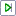将代码运行到特定行并暂停。

• 通过点击“步入”按钮在暂停状态下步入函数和脚本。

• 将断点添加到文件中，以便在运行您的代码时在特定行暂停。

• 编辑器 - 如果文件包含未保存的更改，MATLAB 会在运行文件之前保存该文件。

• 实时编辑器 - MATLAB 运行文件中的所有更改，无论是否保存了它们。

• 命令行窗口 - 如果文件包含未保存的更改，MATLAB 运行文件的已保存版本。您看不到更改结果。

### 显示输出

```n = 50; r = rand(n,1); plot(r) m = mean(r); hold on plot([0,n],[m,m]) hold off title('Mean of Random Uniform Data')```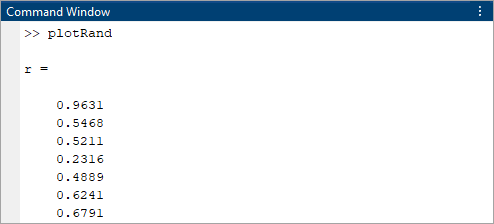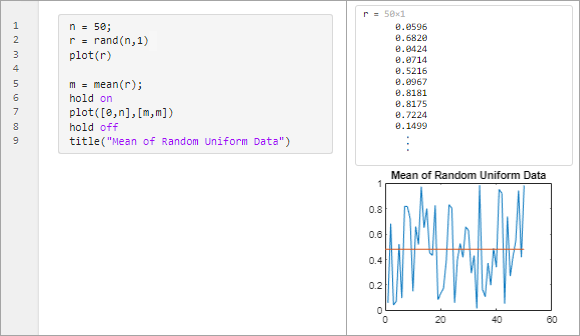### 使用运行到此行进行调试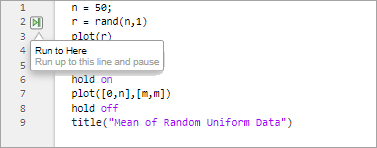• 编辑器实时编辑器选项卡中的按钮更改为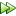按钮。

• 命令行窗口中的提示符将更改为 `K>>`，指示 MATLAB 处于调试模式且键盘受控制。

• MATLAB 使用绿色箭头和绿色突出显示指示暂停的行。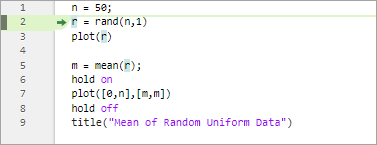MATLAB 会停留在暂停执行的行，直到您继续运行代码为止。要继续运行代码，请点击按钮。MATLAB 会继续运行该文件，直到到达文件末尾或断点。您也可以点击要继续运行到的代码行左侧的“继续运行到此行”按钮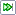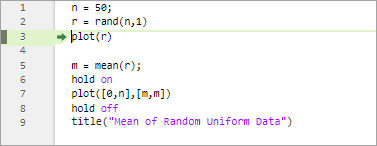### 在调试时查看变量值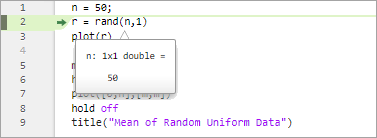### 步入函数

#### 函数调用堆栈### 添加断点并运行代码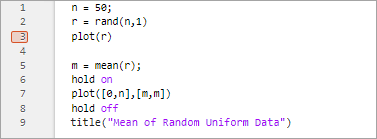• 编辑器实时编辑器选项卡中的按钮更改为按钮。

• 命令行窗口中的提示符将更改为 `K>>`，指示 MATLAB 处于调试模式且键盘受控制。

• MATLAB 使用绿色箭头和绿色突出显示指示暂停的行。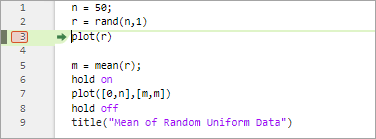### 使用键盘快捷方式或函数进行调试

F5

`dbcont`

F10

（在 macOS 系统上是 Shift+Command+O

`dbstep`

F11

（在 macOS 系统上是 Shift+Command+I

`dbstep in`

Shift+F11

（在 macOS 系统上是 Shift+Command+U

`dbstep out`

Shift+F5

`dbquit`

F12

`dbstop`

F12

`dbclear`